# Master / Apprentice relationship graph in Star Wars

Here’s a graph of master / apprentice relationships for Jedi and Sith in the Star Wars movies. It’s not exhaustive, but it covers the main relationships in Episodes I through VI.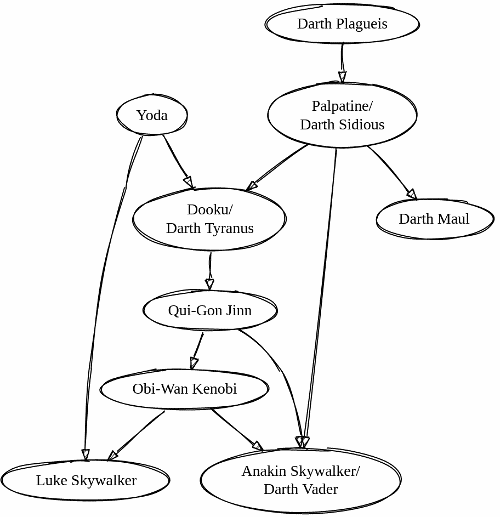Here’s what you get if you add a dashed arrow for who killed whom.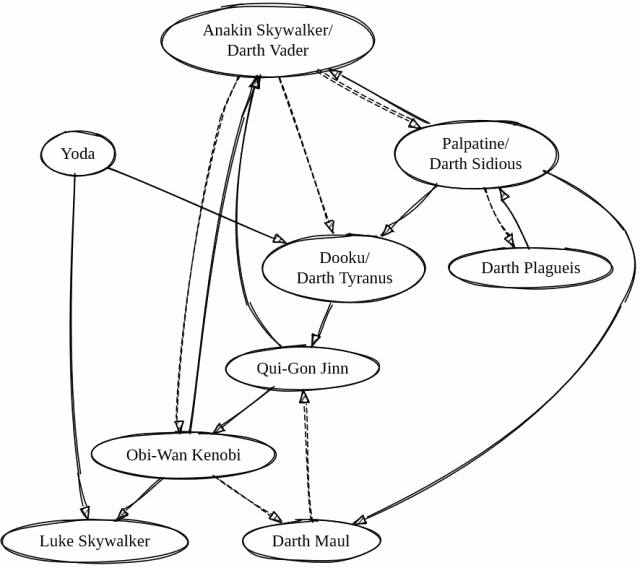Here’s the dot (GraphVis) code that created the diagrams.

digraph G {

Dooku [label="Dooku/\nDarth Tyranus"];
Luke  [label="Luke Skywalker"];
Ben   [label="Obi-Wan Kenobi"];
Liam  [label="Qui-Gon Jinn"];
DS    [label="Palpatine/\nDarth Sidious"]
DP    [label="Darth Plagueis"];
DM    [label="Darth Maul"];

Yoda  -> Dooku;
Yoda  -> Luke;
Dooku -> Liam;
Liam  -> Ben;
Ben   -> Luke;
DS    -> Dooku;
DP    -> DS;
DS    -> DM;

Ben   -> DM [style=dashed];
DM    -> Liam [style=dashed];
DS    -> DP [style=dashed];
}


# Rook graphs and Paley graphs

An m by n rook graph is formed by associating a node with each square of an m by n chess board and connecting two nodes with an edge if a rook can legally move from one to the other. That is, two nodes are connected if they are either in the same row or in the same column.

A Paley graph of order q is a graph with q nodes where q is a prime power and congruent to 1 mod 4. Label each node with an element of the finite field F with q elements and connect two nodes with an edge if the difference between their labels is a quadratic residue. That is, for nodes labeled a and b, connect these nodes if there exist an x in F such that ab = x².

We’ll look at a graph which is both a rook graph and a Paley graph: the 3 by 3 rook graph is the same as the Paley graph of order 9.

## Rook graph 3 x 3

Constructing the rook graph is easy. If we number our 3 by 3 chess board as follows

    1 2 3
4 5 6
7 8 9


then we connect 1 to 2, 3, 4, and 7. We connect 2 to 1, 3, 5, and 8. Etc. This gives us the following graph.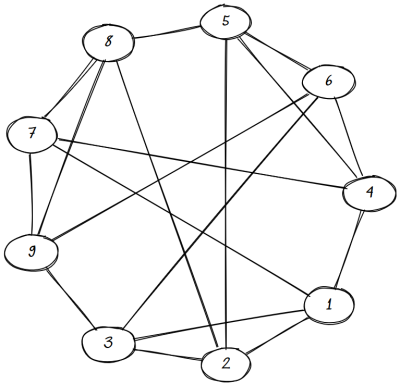(The graph was made with Sketchviz introduced here.)

## Paley graph of order 9

They Paley graph is a little more complicated to construct. First we need to explain what a field with 9 elements is.

A field with 3 elements is just integers mod 3. We can think of the field with 9 elements as the analog of complex numbers: numbers of the form abi where a and b are integers mod 3. As with the complex numbers, i² = -1.

When we square each of the nine elements of our field, we get four distinct non-zero results: 1, 2, i, and 2i. For example, i is one of our squares because

(1 + 2i)(1 + 2i) = (1 – 4) + 4i = i

Here we used -3 = 0 mod 3 and 4 = 1 mod 3.

Since our squares are 1, 2, i, and 2i, that says 0 is connected to these four values. Then we connect 1 to 2, 0, 1 + i and 1 + 2i because each of these numbers minus 1 is a square. If we keep doing this for all nine nodes, we get the following graph.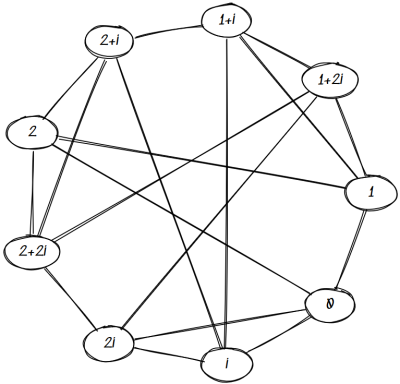## Isomorphism

It’s clear that the two graphs above are relabelings of each other. We can see this explicitly by relabeling our chess board as follows:

    0    i    2i
1  1+i  1+2i
2  2+i  2+2i


Moving horizontally adds i, which is a square, and moving vertically adds 1, which is a square. So the Paley connections are rook connections.

## Uniqueness

Are there more rook graphs that are isomorphic to Paley graphs? No, this is the only one.

Every pair of vertices in a rook graph that are not neighbors have two common. If the points with coordinates (a, b) and (c, d) are on different rows and columns, then both are connected to (a, d) and (c, b).

In a Paley graph of order q, every pair of nodes that aren’t neighbors have (q – 1)/4 common neighbors. For a Paley graph to be isomorphic to a rook graph, we must have (q – 1)/4 = 2, and so q = 9.

# Six degrees of Kevin Bacon, Paul Erdos, and Wikipedia

I just discovered the website Six Degrees of Wikipedia. It lets you enter two topics and it will show you how few hops it can take to get from one to the other.

Since the mathematical equivalent of Six Degrees of Kevin Bacon is Six degrees of Paul Erdős, I tried looking for the distance between Kevin Bacon and Paul Erdős and found this: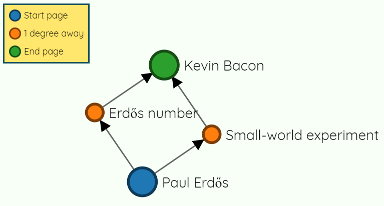Kevin Bacon and Paul Erdős are both known for their prolific collaborations. Many actors have acted with Kevin Bacon, or acted with actors who have acted with him, etc. Many mathematicians wrote papers with Erdős, or have written papers with people who have written papers with him, etc. I’m four degrees of separation from Paul Erdős last I checked.  

Here’s a more complex graph showing the three degrees of separation between Thomas Aquinas and Thomas the Tank Engine.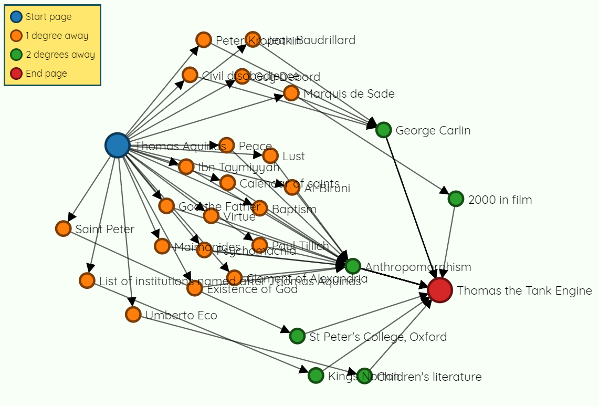Note that the edges are directed, links from the starting page to pages that lead to the target page. If you reverse the starting and ending page, you get different results. For example, there are still three degrees of separation if we reverse our last example, going from Thomas the Tank Engine to Thomas Aquinas, but there are about twice as many possible paths.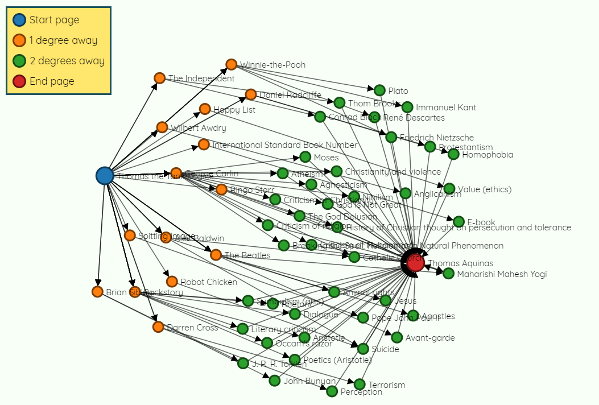## More network posts

 Your Erdős number, the degrees of separation between your and Paul Erdős, can decrease over time because his collaborators are still writing papers. Even Erdős himself continued to publish posthumously because people who began papers with him finished them years later.

# Spectral sparsification

The latest episode of My Favorite theorem features John Urschel, former offensive lineman for the Baltimore Ravens and current math graduate student. His favorite theorem is a result on graph approximation: for every weighted graph, no matter how densely connected, it is possible to find a sparse graph whose Laplacian approximates that of the original graph. For details see Spectral Sparsification of Graphs by Dan Spielman and Shang-Hua Teng.

You could view this theorem as a positive result—it’s possible to find good sparse approximations—or a negative result—the Laplacian doesn’t capture very well how densely a graph is connected.

Related: Blog posts based on my interview with Dan Spielman at the 2014 Heidelberg Laureate Forum.

# Random minimum spanning trees

I just ran across a post by John Baez pointing to an article [the link has gone away] by Alan Frieze on random minimum spanning trees.

Here’s the problem.

1. Create a complete graph with n nodes, i.e. connect every node to every other node.
2. Assign each edge a uniform random weight between 0 and 1.
3. Find the minimum spanning tree.
4. Add up the edges of the weights in the minimum spanning tree.

The surprise is that as n goes to infinity, the expected value of the process above converges to the Riemann zeta function at 3, i.e.

ζ(3) = 1/1³ + 1/2³ + 1/3³ + …

Incidentally, there are closed-form expressions for the Riemann zeta function at positive even integers. For example, ζ(2) = π² / 6. But no closed-form expressions have been found for odd integers.

## Simulation

Here’s a little Python code to play with this.

    import networkx as nx
from random import random

N = 1000

G = nx.Graph()
for i in range(N):
for j in range(i+1, N):

T = nx.minimum_spanning_tree(G)
edges = T.edges(data=True)

print( sum( e["weight"] for e in edges ) )


When I ran this, I got 1.2307, close to ζ(3) = 1.20205….

I ran this again, putting the code above inside a loop, averaging the results of 100 simulations, and got 1.19701. That is, the distance between my simulation result and ζ(3) went from 0.03 to 0.003.

There are two reasons I wouldn’t get exactly ζ(3). First, I’m only running a finite number of simulations (100) and so I’m not computing the expected value exactly, but only approximating it. (Probably. As in PAC: probably approximately correct.) Second, I’m using a finite graph, of size 1000, not taking a limit as graph size goes to infinity.

My limited results above suggest that the first reason accounts for most of the difference between simulation and theory. Running 100 replications cut the error down by a factor of 10. This is exactly what you’d expect from the central limit theorem. This suggests that for graphs as small as 1000 nodes, the expected value is close to the asymptotic value.

You could experiment with this, increasing the graph size and increasing the number of replications. But be patient. It takes a while for each replication to run.

## Generalization

The paper by Frieze considers more than the uniform distribution. You can use any non-negative distribution with finite variance and whose cumulative distribution function F is differentiable at zero. The more general result replaces ζ(3) with ζ(3) / F ‘(0). We could, for example, replace the uniform distribution on weights with an exponential distribution. In this case the distribution function is 1 – exp(-x), at its derivative at the origin is 1, so our simulation should still produce approximately ζ(3). And indeed it does. When I took the average of 100 runs with exponential weights I got a value of 1.2065.

There’s a little subtlety around using the derivative of the distribution at 0 rather than the density at 0. The derivative of the distribution (CDF) is the density (PDF), so why not just say density? One reason would be to allow the most general probability distributions, but a more immediate reason is that we’re up against a discontinuity at the origin. We’re looking at non-negative distributions, so the density has to be zero to the left of the origin.

When we say the derivative of the distribution at 0, we really mean the derivative at zero of a smooth extension of the distribution. For example, the exponential distribution has density 0 for negative x and density exp(-x) for non-negative x. Strictly speaking, the CDF of this distribution is 1 – exp(-x) for non-negative x and 0 for negative x. The left and right derivatives are different, so the derivative doesn’t exist. By saying the distribution function is simply exp(-x), we’ve used a smooth extension from the non-negative reals to all reals.

# Visualizing graph spectra like chemical spectra

You can associate a matrix with a graph and find the eigenvalues of that matrix. This gives you a spectrum associated with the graph. This spectrum tells you something about the graph analogous to the way the spectrum of a star’s light tells you something about the chemical composition of the star.

So maybe it would be useful to visualize graph spectra the way you visualize chemical spectra. How could you do this?

First, scale the spectrum of the graph to the visual spectrum. There are different kinds of matrices you can associate with a graph, and these have different natural ranges. But if we look at the spectrum of the Laplacian matrix, the eigenvalues are between 0 and n for a graph with n nodes.

Eigenvalues typically correspond to frequencies, not wavelengths, but chemical spectra are often displayed in terms of wavelength. We can’t be consistent with both, so we’ll think of our eigenvalues as wavelengths. We could easily redo everything in terms of frequency.

This means we map 0 to violet (400 nm) and n to red (700 nm). So an eigenvalue of λ will be mapped to 400 + 300 λ/n. That tells us where to graph a spectral line, but what color should it be? StackOverflow gives an algorithm for converting wavelength to RGB values. Here’s a little online calculator based on the same code.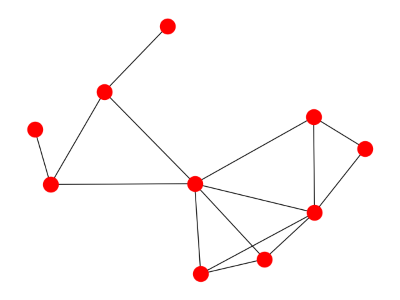Here’s what the spectrum looks like:Here’s a cyclic graph: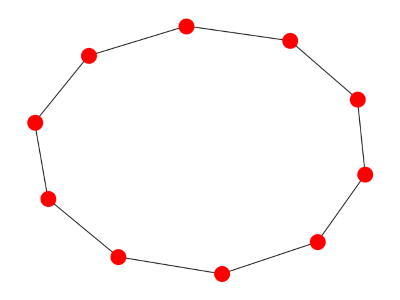And here’s its spectrum:Finally, here’s a star graph: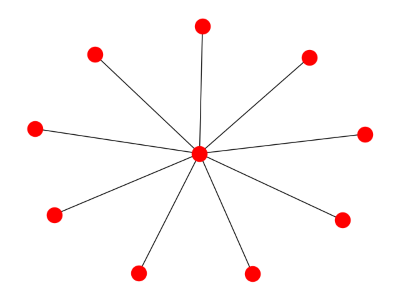And its spectrum:Related: Ten spectral graph theory posts

# Measuring graph robustness

There are a couple ways to measure how well a graph remains connected when nodes are removed. One ways is to consider nodes dropping out randomly. Another way, the one we look at here, assumes an adversary is trying to remove the most well-connected nodes. This approach was defined by Schneider et al . It is also described, a little more clearly, in .

The robustness of a graph is defined asThen  explains that

N is the total number of nodes in the initial network and S(q) is the relative size of the largest connected component after removing q nodes with the highest degrees. The normalization factor 1/N ensures 1/NR ≤ 0.5. Two special cases exist: R = 1/N corresponds to star-like networks while R = 0.5 represents the case of the fully connected network.

Apparently “relative size” means relative to the size of the original network, not to the network with q nodes removed.

There is one difference between  and . The former defines the sum up to N and the latter to N-1. But this doesn’t matter because S(N) = 0: when you remove N nodes from a graph with N nodes, there’s nothing left!

I have a couple reservations. One is that it’s not clear whether R is well defined.

Consider the phrase “removing q nodes with the highest degrees.” What if you have a choice of nodes with the highest degree? Maybe all nodes have degree no more than n and several have degree exactly n. It seems your choice of node to remove matters. Removing one node of degree n may give you a very different network than removing another node of the same degree.

Along the same lines, it’s not clear whether it matters how one removes more than one degree. For S(2), for example, does it matter whether I remove the two most connected nodes from the original graph at once, or remove the most connected node first, then the most connected node from what remains?

My second reservation is that I don’t think the formula above gives exactly the extreme values it says. Start with a star graph. Once you remove the center node, there are only isolated points left, and each point is 1/N of the original graph. So all the S(q) are equal to 1/N. Then R equals (N – 1)/ N2, which is less than 1/N.

With the complete graph, removing a point leaves a complete graph of lower order. So S(q) = (Nq)/N. Then R equals (N – 1)/2N, which is less than 1/2.

***

 CM Schneider et al, Mitigation of malicious attacks on networks. P. Natl. Acad. March 8, 2011, vol. 108. no. 10

 Big Data of Complex Networks, CRC Press. Matthias Dehmer et al editors.

# Cover time of a graph: cliques, chains, and lollipops

## Cover time

The cover time of a graph is the expected time it takes a simple random walk on the graph to visit every node. A simple random walk starts at some node, then at each step chooses with equal probability one of the adjacent nodes. The cover time is defined to be the maximum over all starting points of expected time to visit all nodes.

It seems plausible that adding edges to a graph would decrease its cover time. Sometimes this is the case and sometimes it isn’t, as we’ll demonstrate below.

## Chains, complete graphs, and lollipops

This post will look at three kinds of graphs

1. a chain of  n vertices
2. a complete graph on n vertices
3. a “lollipop” with n vertices

A chain simply connects vertices in a straight line. A complete graph connects every node to every other node.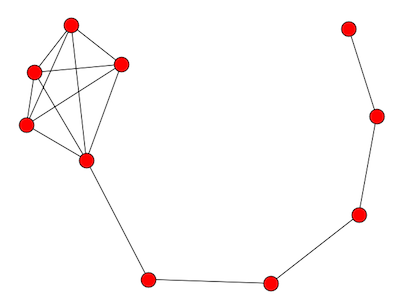A lollipop with n vertices takes a chain with n/2 vertices and complete graph on n/2 vertices and joins them together. The image above is a lollipop graph of order 10. The complete graph becomes a clique in the new graph.

The image looks more like a kite than a lollipop because that’s the way my software (networkx) drew it by default. If you’d like, image the complete graph more round and the chain more straight so that the graph more closely resembles a lollipop.

## Random walks on graphs

Chains have a long cover time. Complete graphs have a short cover time. What about lollipops?

A plausible answer would be that a lollipop graph would have a moderate cover time, somewhere between that of a complete graph and a chain. But that’s not the case. In fact, the lollipop has a longer cover time than either the complete graph or the chain.

You could think of a lollipop graph with n vertices as a chain of length n with extra edges added. This shows that adding edges does not always decrease the cover time.

The cover times for the three graphs we consider here are of different orders: O(n log n) for the complete graph, O(n2) for the chain, and O(n3) for the lollipop.

Now these are only asymptotic results. Big-O notation leaves out proportionality constants. We know that for sufficiently large n the cover times are ordered so that the complete graph is the smallest, then the chain, then the lollipop. But does n have to be astronomically large before this happens? What happens with a modest size n?

There’s a theoretical result that says the cover time is bounded by 2m(n-1) where m is the number of edges and n the number of vertices. This tells us that when we say the cover time for the chain is O(n2) we can say more, that it’s less than 2n2, so at least in this case the proportionality constant isn’t large.

We’ll look at the cover times with some simulation results below based on n = 10 and n = 30 based on 10,000 runs.

With 10 nodes each, the cover times for the complete graph, chain, and lollipop were 23.30, 82.25, and 131.08 respectively.

With 30 nodes the corresponding cover times were 114.37, 845.16, and 3480.95.

So the run times were ordered as the asymptotic theory would suggest.

# Rapidly mixing random walks on graphs

Random walks mix faster on some graphs than on others. Rapid mixing is one of the ways to characterize expander graphs.

By rapid mixing we mean that a random walk approaches its limiting (stationary) probability distribution quickly relative to random walks on other graphs.

Here’s a graph that supports rapid mixing. Pick a prime p and label nodes 0, 1, 2, 3, …, p-1. Create a circular graph by connecting each node k to k+1 (mod p).  Then add an edge between each non-zero k to its multiplicative inverse (mod p). If an element is its own inverse, add a loop connecting the node to itself. For the purpose of this construction, consider 0 to be its own inverse. This construction comes from .

Here’s what the graph looks like with p = 23.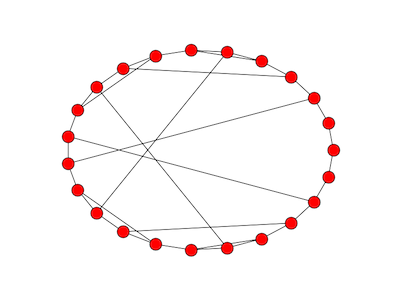This graph doesn’t show the three loops from a node to itself, nodes 1, 0, and 22.

At each node in the random walk, we choose an edge uniformly and follow it. The stationary distribution for a random walk on this graph is uniform. That is, in the long run, you’re equally likely to be at any particular node.

If we pick an arbitrary starting node, 13, and take 30 steps of the random walk, the simulated probability distribution is nearly flat.By contrast, we take a variation on the random walk above. From each node, we move left, right, or stay in place with equal probability. This walk is not as well mixed after 100 steps as the original walk is after only 30 steps.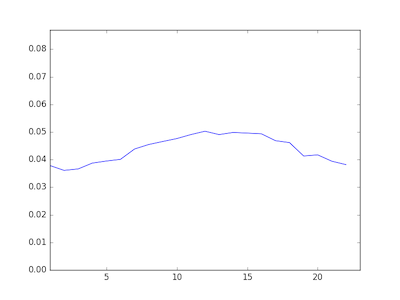You can tell from the graph that the walk started around 13. In the previous graph, evidence of the starting point had vanished.

The code below was used to produce these graphs.

To investigate how quickly a walk on this graph converges to its limiting distribution, we could run code like the following.

    from random import random
import numpy as np
import matplotlib.pyplot as plt
m = 23

# Multiplicative inverses mod 23
inverse = [0, 1, 12, 8, 6, 14, 4, 10, 3, 18, 7, 21, 2, 16, 5, 20, 13, 19, 9, 17, 15, 11, 22]

# Verify that the inverses are correct
assert(len(inverse) == m)
for i in range(1, m):
assert (i*inverse[i] % 23 == 1)

# Simulation
num_reps = 100000
num_steps = 30

def take_cayley_step(loc):
u = random() * 3
if u < 1: # move left
newloc = (loc + m - 1) % m
elif u < 2: # move right
newloc = (loc + 1) % m
else:
newloc = inverse[loc] # or newloc = loc
return newloc

stats = *m
for i in range(num_reps):
loc = 13 # arbitrary fixed starting point
for j in range(num_steps):
loc = take_cayley_step(loc)
stats[loc] += 1

normed = np.array(stats)/num_reps
plt.plot(normed)
plt.xlim(1, m)
plt.ylim(0,2/m)
plt.show()


## Related posts

* * *

 Shlomo Hoory, Nathan Linial, Avi Wigderson. “Expander graphs and their applications.” Bulletin of the American Mathematical Society, Volume 43, Number 4, October 2006, pages 439–561.

# Ten spectral graph theory posts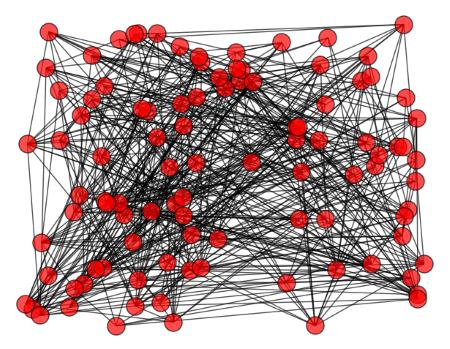Here are 10 blog posts I wrote earlier this year about spectral graph theory, studying graphs via the eigenvalues of matrices associated with the graphs.